# Machine level representation of data

Programming China 2021-10-14 03:04:14

Representation of unsigned and signed numbers

Fixed point and floating point representations of numbers

Character and Chinese character coding method

Data check code

# Numerical data represent

data ： Signed number An unsigned number

Signed number ： Original code Complement code Inverse code

• Numerical data in a computer

Binary number （B

Octal number （Q

Decimal number （D

• Unsigned and signed numbers

An unsigned number ： All binary bits of the whole machine word length represent numeric bits , Equal to the absolute value of a number

The machine word length is n+1 The representation range of an unsigned number of bits 0~2n+1-1

Signed number ： just 、 negative

The machine word length is n+1 The signed number of bits represents the range -2n+1~2n+1-1 8 position （-128-127

Integers ： Sign bit Value bits .

decimal ： Sign bit . Value bits

• Original code representation
 Positive numbers 0|X| negative 1|X| special 0.0110 0.0110 -0.0110 1.0110 +0 00000 0110 0110 -0110 10110 -0 10000 +0 0.0000 -0 1.0000
• Reverse code representation
 Positive numbers = Original code negative 1 |X| Take the opposite special 0.0110 0.0110 -0.0110 1.1001 +0 00000 0110 00110 -0110 11001 -0 11111 +0 0.0000 -0 1.1111
• Complement representation
 Positive numbers = Original code negative 1 |X| Take the opposite +1 special 0.0110 0.0110 -0.0110 1.1010 +0 00000 0110 00110 -0110 11010 -0 00000 +0 0.0000 -0 0.0000

The original code and complement are interchangeable

X Another way to be negative ： The first of the last 1 And to the right 0 remain unchanged , On the left, take the opposite , Sign bit

remain unchanged .

[X] primary =10111001100

[X] back =11000110100

• Comparison of three coding systems

Complement and inverse sign bits can be treated as part of numeric bits , Operate with numeric bits ; However, the sign bit of the original code is not allowed to operate with the numeric bit , It has to be dealt with separately

# Fixed point representation and floating point representation of machine numbers -- According to whether the position of the decimal point is fixed

## Fixed point representation ： The position of all decimal points is fixed

• Fixed point decimals ：Xs.X1X2……Xn

Scope of representation X Maximum positive number =1-2-n   X Minimum positive number =2-n

Original code ：-1-2-n~1-2-n

Complement code ：-1~1-2-n

• Fixed point integers ：XsX1X2……Xn ( The machine word length has n+1 position ）

The original code indicates the range ：-2n-1~2n-1

The complement indicates the range ：-2n~2n-1

## Floating point representation

Floating point numbers ： The position of the decimal point floats as needed

N=M×rE

1. r Cardinal number , Usually r=2
2. E It's a step code , Signed integers , It is often expressed by code shift or complement
3. M mantissa , Signed decimal number , It is often expressed by original code or complement code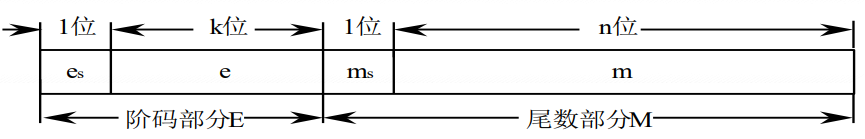• The bottom of a floating point number is implicit , Does not appear in the whole number of machines
• The sign bit of the order code is es, Order code e The size of is reflected in the number N The actual position of the decimal point in
• The sign bit of mantissa is ms, It is the sign bit of the entire floating point number , mantissa m Represents the precision of the floating point number

### The representation range of floating-point numbers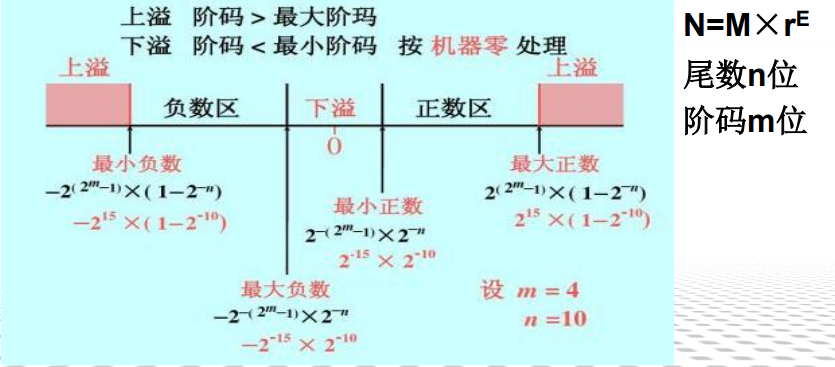• Normalize floating-point numbers ： In order to improve the operation accuracy , We need to make full use of the mantissa significand . That is, the highest digit of the mantissa must be a valid value .

1/2<=|M|<1

• When the mantissa is represented by a complement , The normalized floating-point number shall satisfy that the highest digit of the mantissa is different from the sign bit
 Truth value Order code （E) mantissa （M） The absolute value is the maximum negative number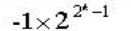01111 1.0000 The absolute value is the smallest negative number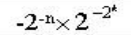10000 1.1111 Normalized absolute minimum negative number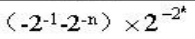1000 1.0111 Maximum positive number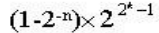01111 0.1111 Minimum positive number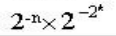10000 0.0001 Normalized minimum positive number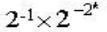10000 0.1000

## Code shifting representation

In truth X Add an offset value （ constant ）, amount to X On the number axis, I shifted a distance in the positive direction .

• [X] move = Offset value +X
• Offset value =2n-1

characteristic ：

• highest “0” A negative number , highest “1” It means a positive number
• Shift to full 0 when , His corresponding truth value is the smallest , Shift to full 1 when , The corresponding truth value is the maximum
• The representation of truth zero is unique in code shift ,1000 0000
• The shift maps the truth value to a positive field , Therefore, the code shift can be regarded as an unsigned number , Compare sizes directly according to the unsigned number rule
• The code shift and complement of the same value are opposite except the highest bit , Everyone else is the same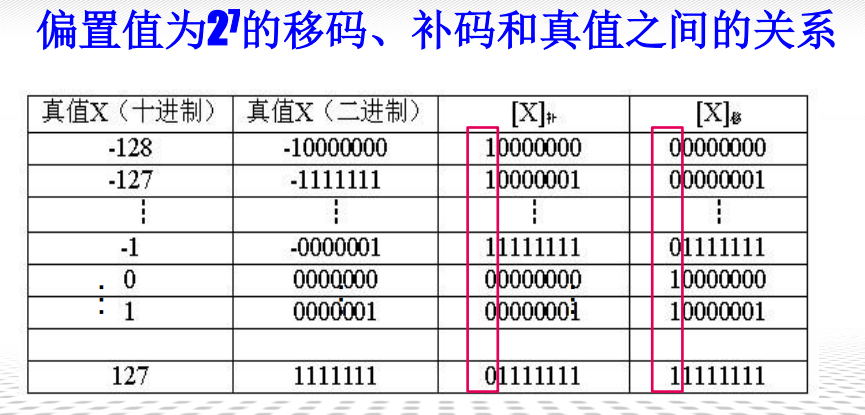The reason why order code adopts code shift

• The reason why the order code of floating point number adopts code shift , If the order code is large, its corresponding truth value is large , If the order code is small, its corresponding truth value is small
• Simplify the zero judgment circuit in the machine . When the order codes are all zero （ Everyone who shifts the code is 0 when , The corresponding order code value is the smallest ）, The mantissa is all zero , Indicates that the machine is zero

## Fixed point 、 Floating point representation and fixed point representation 、 Floating point computer

### Fixed point 、 The difference between floating point notation

1. If the word length is the same , The range represented by floating-point numbers is much larger than that of fixed-point numbers
2. Floating point precision decreases , The points on the number axis are arranged more sparsely
3. Floating point operations are more complex than fixed-point operations
4. Overflow handling ： Fixed point operation , The result of the operation is beyond the representation range of the number , There is an overflow , But in floating-point operations , The operation result exceeds the representation range of the mantissa and does not necessarily overflow , Overflow occurs when the order code exceeds the range that can be represented .

### Fixed point machine and floating point machine

• Pointing machine ： Mainly fixed-point operation , Floating point operation is realized by software
• Pointing machine + Floating point software ： Floating point arithmetic unit is a unit specially used for floating point arithmetic
• Floating point machine ： With floating-point instructions and basic floating-point arithmetic unit

# The representation of non numerical data

Non numeric data is character data , That is, the characters 、 character string 、 Various data such as image symbols and Chinese characters , It is not used to represent the size of the value

## Representation of characters and strings

1. ASCII Character encoding

Represent a character in seven binary digits , Including ten binary digits （0~9）、52 English capital letters （A-Z,a-z）、34 A special symbol ,3 A control symbol , common 128 Characters

A computer usually stores one character per byte

1. String storage

Vector storage method ： Occupy a contiguous space in memory , Each byte stores a character code , All elements of the string are physically adjacent . What is actually stored in each byte is the of the corresponding character ASCII code .

## Unified code

Unicode： Unified code , A character coding scheme that can accommodate all characters and symbols in the world .

Unicode The basic method ： Use one 16 A number of bits to represent each symbol , Can be said 65536 A different character or symbol . It is called the Basic Multilingual plane （BMP）, namely UCS-2 code .

USC-4, Can be said 100 More than 10000 custom characters

# Representation of decimal and number strings

## Coding of decimal numbers

Use four binary numbers to represent one decimal number , Called binary coded decimal numbers , abbreviation BCD code .

BCD The code has a binary form , It retains the characteristics of decimal numbers

 Decimal system 8421 code 2421 code 0 0000 0000 0011 1 0001 0001 0100 2 0010 0010 0101 3 0011 0011 0110 4 0100 0100 0111 5 0101 1011 1000 6 0110 1100 1001 7 0111 1101 1010 8 1000 1110 1011 9 1001 1111 1100

## Decimal string

1. Uncompressed decimal string ： A byte that holds a decimal number or symbol ASCII-7 code （ A byte =8 Bit binary number 1Byte=8bit）
2. Compressed decimal string ： One byte can hold two bits BCD A decimal number represented by a code , Save storage space , Convenient for direct decimal arithmetic operation

# Examples of data representation in modern microcomputer systems

Most modern microcomputer systems use Intel Series microprocessors , structure ：IA-32 structure

IA-32 Structure basic data type ： byte （8 position ）、 word （16 position ）、 Two words （32 position ）、 Four words （64 position ）、 Double four characters （128 position ）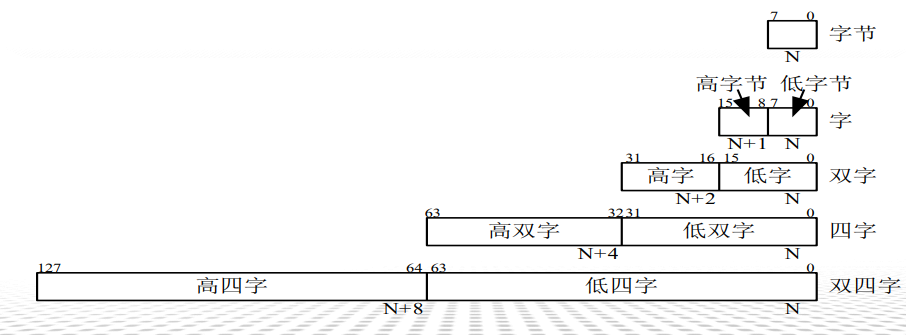1. Unsigned integer ：
1. byte ：0~255
2. word ：0~65535
3. Two words ：0~2^32-1
4. Four words ：0~2^64-1
2. Signed integers
1. byte ：-128~127
2. word ：-32768~32767
3. Two words ：-2^31~2^31-1
4. Four words ：-2^63~2^63-1
3. Floating point numbers

And IEEE 754 The format specified in the standard directly corresponds to

1. The pointer is the address of the main memory unit ,IA-32 Structure defines two types of pointers ： Near pointer （32 position ） And the far pointer （64 position ）
2. String data ： Including bit string 、 Byte string 、 String and double string . A string can contain from one byte to 4GB The content of .
3. BCD Count ：IA-32 In structure BCD Code actually means 8421 code
Please bring the original link to reprint ,thank